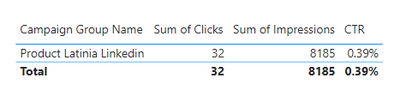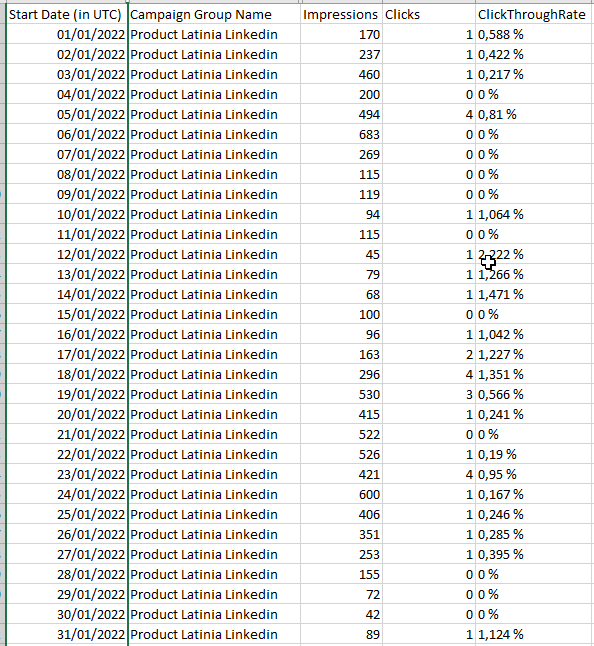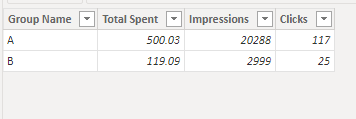cancel
Showing results for
Did you mean:New Member

## Average between two columns not correct

Hello,

I've been trying to calculate the average of the division of 2 columns in PowerBi Desktop and not receive the right results.As you can see the column CTR is returning 0,66% for the first line, when it should be: 0.58%

And it return 0,80 for the second, when it should be 0,83.

My Column CTR is :

1 ACCEPTED SOLUTIONCommunity Support

Hi @jhlatinia ,

The result you get is incorrect is because it is the sum of all rows. In other words, they calculate the percentage of each row first and then sum it, which gives a different result than summing and then calculating the percentage.

``````CTR =
VAR _a =
SUMMARIZE (
'Table',
'Table'[Campaign Group Name],
"CTR",
DIVIDE (
CALCULATE (
SUM ( 'Table'[Clicks] ),
FILTER (
'Table',
[Campaign Group Name] = EARLIER ( 'Table'[Campaign Group Name] )
)
),
CALCULATE (
SUM ( 'Table'[Impressions] ),
FILTER (
'Table',
[Campaign Group Name] = EARLIER ( 'Table'[Campaign Group Name] )
)
)
)
)
RETURN
AVERAGEX ( _a, [CTR] )
``````

Final output:Best Regards,

Jianbo Li

If this post helps, then please consider Accept it as the solution to help the other members find it more quickly.

7 REPLIES 7Community Support

Hi @jhlatinia ,

The result you get is incorrect is because it is the sum of all rows. In other words, they calculate the percentage of each row first and then sum it, which gives a different result than summing and then calculating the percentage.

``````CTR =
VAR _a =
SUMMARIZE (
'Table',
'Table'[Campaign Group Name],
"CTR",
DIVIDE (
CALCULATE (
SUM ( 'Table'[Clicks] ),
FILTER (
'Table',
[Campaign Group Name] = EARLIER ( 'Table'[Campaign Group Name] )
)
),
CALCULATE (
SUM ( 'Table'[Impressions] ),
FILTER (
'Table',
[Campaign Group Name] = EARLIER ( 'Table'[Campaign Group Name] )
)
)
)
)
RETURN
AVERAGEX ( _a, [CTR] )
``````

Final output:Best Regards,

Jianbo Li

If this post helps, then please consider Accept it as the solution to help the other members find it more quickly.Community Support

Hi @jhlatinia ,

What does your Start Date look like? What is the relationship between the date and the value?

Sorry for that the information you have provided is not making the problem clear to me. Can you please share more details to help us clarify your scenario?

Refer to:

How to provide sample data in the Power BI Forum

Best Regards,

Jianbo Li

If this post helps, then please consider Accept it as the solution to help the other members find it more quickly.New Member

Hi,This is the format.Community Support

Hi @jhlatinia ,

Are you trying to calculate the average of the CTR?

Here is my sample:``````Measure =
VAR _a =
SUMMARIZE (
"CTR",
DIVIDE (
CALCULATE (
),
CALCULATE (
)
)
)
RETURN
AVERAGEX ( _a, [CTR] )
``````

Final output:Best Regards,

Jianbo Li

If this post helps, then please consider Accept it as the solution to help the other members find it more quickly.New Member

The measure look right, may I had, that Impressions or clicks are the sum of each lines of the last 7 days.

Meaning, I filtered by Start Date which doesn't show in the image, does it change the measure?

Sorry I'm quite new to all of this! And thank you for your help, really appriciated :).Super User

@jhlatinia Are Impressions and Clicks measures? If so, what are the formulas?

Become an expert!: Enterprise DNA
External Tools: MSHGQM
Latest book!:
Mastering Power BI 2nd EditionDAX is easy, CALCULATE makes DAX hard...New Member

@Greg_Deckler, no they are normal number column data.Announcements#### Exclusive opportunity for Women!

Join us for a free, hands-on Microsoft workshop led by women trainers for women where you will learn how to build a Dashboard in a Day!#### Power Platform Conference-Power BI and Fabric Sessions

Join us Oct 1 - 6 in Las Vegas for the Microsoft Power Platform Conference.Top Solution Authors
Top Kudoed Authors
Users online (3,269)